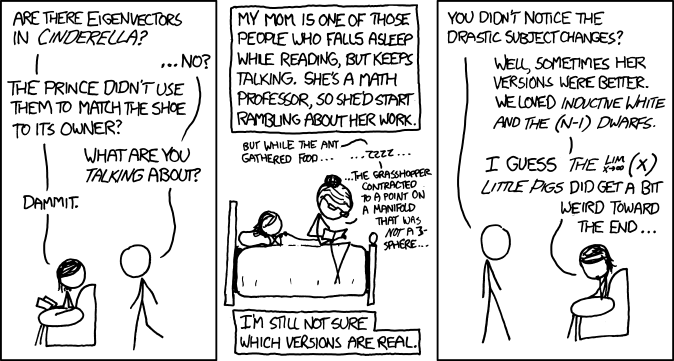# April, 2015Archive

Apr 13

## Numerical Word Search

Posted in Comic Strips,

The FoxTrot Strip from November 6, 2005 contained a “numerical word search”

You can find the strip here.

There are seven clues which are arithmetic problems, but there is also the derivative of 43981 x , the definite integral of x^3 from 0 to 16, and the sum from k equals 0 to 47 of k squared.    I asked my calculus students to perform all these calculations.

Apr 13

## Limits and Fairy Tales

Posted in Comic Strips,

I asked my calculus students to read this xkcd strip from http://xkcd.com/872/a) What is $\lim_{x\rightarrow \infty} (x )$?
b) Explain what might have been weird about the $\lim_{x\rightarrow \infty} (x )$ little pigs.